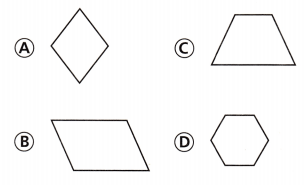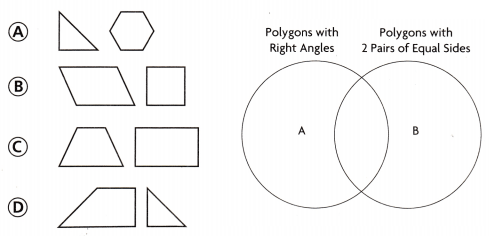# Texas Go Math Grade 3 Lesson 15.3 Answer Key Classify Plane Figures

Refer to our Texas Go Math Grade 3 Answer Key Pdf to score good marks in the exams. Test yourself by practicing the problems from Texas Go Math Grade 3 Lesson 15.3 Answer Key Classify Plane Figures.

## Texas Go Math Grade 3 Lesson 15.3 Answer Key Classify Plane Figures

Unlock the Problem

A Venn diagram shows how sets of things are related. In the Venn diagram at the right, one circle has figures that are rectangles. Figures that are rhombuses are in the other circle. The figures in the section where the circles overlap are both rectangles and rhombuses.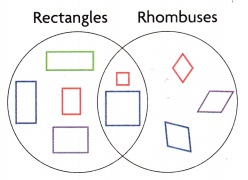What type of quadrilateral is in both circles?

What do I need to find?

What information am I given?

the circles labeled ________ and _________

Plan

What is my plan or strategy?

Solve

Which quadrilaterals have 2 pairs of opposite sides that are parallel?

Which quadrilaterals have 4 sides of equal length?

Which quadrilaterals have 4 right angles?

The quadrilaterals in the section where the circles overlap have _________ pairs of opposite sides that are parallel, _________ sides of equal length, and ________ right angles.

So, ________ are in both circles.
What type of quadrilateral is in both circles?
Squares , Rhombus and Rectangle

What do I need to find?
The section where the circles overlap are both rectangles and rhombuses.

What information am I given?
Squares , Rhombus and Rectangle are the quadrilaterals
The circles labeled Rectangles and Rhombuses

Plan

What is my plan or strategy?
Squares , Rhombus and Rectangle are the quadrilaterals

Solve

All quadrilaterals have the four corners and four sides

Which quadrilaterals have 2 pairs of opposite sides that are parallel?
Squares and rectangles have 2 pairs of opposite sides that are parallel

Which quadrilaterals have 4 sides of equal length?
Squares have 4 sides of equal length.

Which quadrilaterals have 4 right angles?
Squares and rectangles have 4 right angles

The quadrilaterals in the section where the circles overlap have 2 pairs of opposite sides that are parallel, 4 sides of equal length, and 4 right angles.

So, Squares are in both circles.

Try Another Problem

The Venn diagram at the right shows the figures Abbie used to make a picture. Where would the figure shown below be placed in the Venn diagram?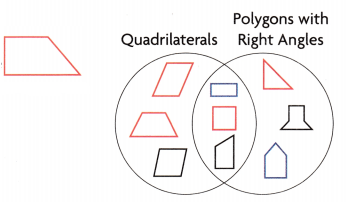What do I need to find?

What information am I given?

Plan

What is my plan or strategy?

Solve
The figure is placed at polygons with right angle
What do I need to find?
The figure shown in right side where it fit

What information am I given?
The information is about the quadrilaterals and the polygons with right angles

Plan

What is my plan or strategy?
To show a quadrilateral with right angle.

Question 1.
How many figures do not have right angles?
Explanation:
3 figures do not have right angles

Question 2.
How many red figures have right angles but are not quadrilaterals?
Explanation:
Triangle have right angles but are not quadrilaterals

Question 3.
Analyze What is a different way to sort the figures?
Answer: Parallel  with one pair of opposite sides and 2 pair of opposite sides
Explanation:
different way to sort the figures
Parallel  with one pair of opposite sides and 2 pair of opposite sides

Math Talk
Mathematical Processes

What name can be used to describe all the figures in the Venn diagram? Explain how you know.
Explanation:
Every shape has more than 2 angles.

Share and Show

Use the Venn diagram for 1-2.Question 1.
Jordan is sorting the figures at the right in a Venn diagram. Where does the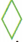go?
First, look at the sides and angles of the polygons.
Next, draw the polygons in the Venn diagram below.
The figure above has ________ sides of equal length and _________ right angles.
So, the figure goes in the circle labeled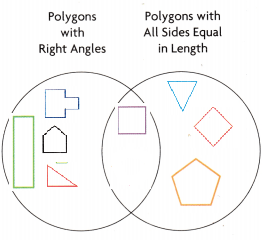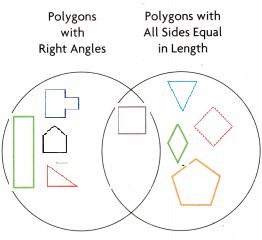it is placed in polygons with all sides equal in length
The figure above has 4 sides of equal length and 0 right angles.

Question 2.
Where in the Venn diagram would you place a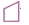?is placed in polygons with right angles

Problem Solving

Question 3.
H.O.T. Multi-Step Use Math Language Eva drew the Venn diagram at the right. What labels could she have used for the diagram? Where would each label go?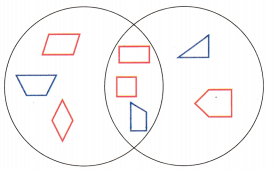Explanation:
The labels are polygons without right angles and
polygons with right angles

Question 4.
H.O.T. Multi-Step Display Draw and label a Venn diagram to show one way you can sort a parallelogram, a rectangle, a square, a trapezoid, and a rhombus.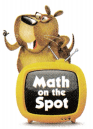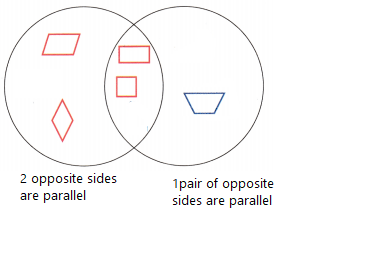Explanation:
Drawn and labeled a Venn diagram to show one way that we can sort a parallelogram, a rectangle, a square, a trapezoid, and a rhombus

Fill in the bubble for the correct answer choice.

Question 5.
Max classifies the street signs he sees by shape, and then sorts them in a Venn diagram. The circles are labeled ‘Polygons with Right Angles” and “Polygons with All Sides Equal in Length.” Which sign is in the section where the two circles overlap?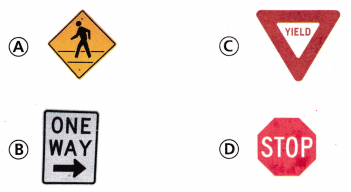Explanation:
‘Polygons with Right Angles” and “Polygons with All Sides Equal in Length.” shows the option B

Question 6.
Multi-Step Jenna makes a design using pattern blocks. Which pattern block is placed incorrectly in the Venn diagram?Explanation:
Option D is placed wrong because the opposite sides only one side is parallel

Texas Test Prep

Question 7.
What label could describe Circle A?(A) Polygons with 4 Right Angles
(B) Polygons with 2 Pairs of Opposite Sides That Are Parallel
(C) Polygons with 2 Pairs of Sides of Equal Length
(D) Polygons with All Sides of Equal Length
Explanation:
Circle A describes Polygons with All Sides of Equal Length

### Texas Go Math Grade 3 Lesson 15.3 Homework and Practice Answer Key

Use the Venn diagram for 1 and 2.

Question 1.
Draw the polygons in the Venn diagram.Explanation:
Polygons are separated according to polygons with parallel sides
and polygons with right angles

Question 2.
Where in the Venn diagram would you place a?
Explanation: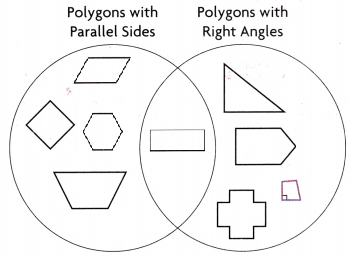Problem Solving

Question 3.
Tia wants to include the figures at the right in one circle of a Venn diagram. What label can she use?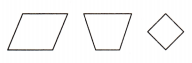Answer: Parallelogram with one pair of opposite sides are equal
Explanation:
The parallelogram, Trapezoid and rhombus have one pair of opposite sides are equal

Lesson Check

Question 4.
Fran draws a Venn diagram with two circles and labels the circles “Polygons with Parallel Sides” and “Quadrilaterals with Parallel Sides.” Which figure belongs only in the circle labeled “Polygons with Parallel Sides?”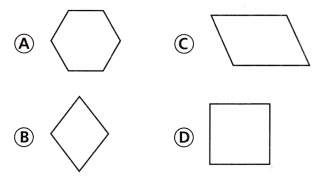Explanation:
Option A belongs only in the circle labeled “Polygons with Parallel Sides

Question 5.
Sean draws a Venn diagram with two circles and labels the circles “Polygons with 4 Equal Sides” and “Polygons with Parallel Sides.” Which figure can he draw where the circles overlap?